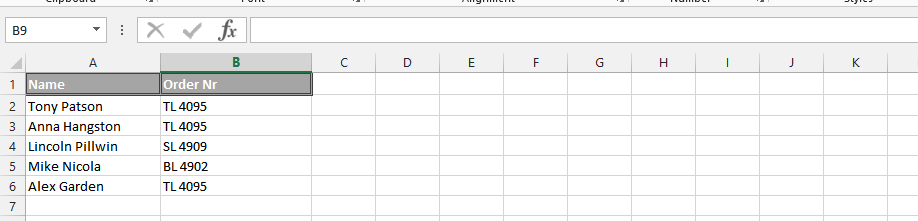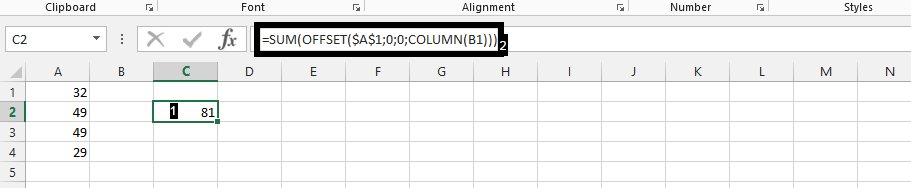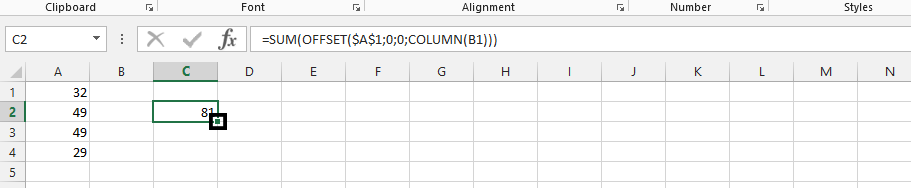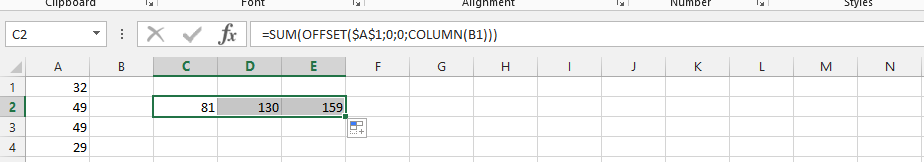#### How to Increment Row Number in Excel Formula

The increment of row number in a formula has to do with the functions that are utilized in the successfulness of increment.

But first, we need data like this:## Formula preparation

You should click on an empty cell (1), and then type in this formula using SUM, OFFSET and COLUMN together in one formula: =SUM(OFFSET(\$A\$1;0;0;COLUMN(B1))) (2).## Increasing row numbers

Proof that there is a success in the increment by clicking on the small square that shows on lower-right corner of the dark green, and drag it to left.Note: It is better to stick to 3 because after the third it will show the same number over and over again.## ROW function

To increment the row number in an Excel formula, you can use the ROW() function, which returns the row number of the current cell. You can then use this value in your formula to reference cells in other rows.

For example, if you want to sum the values in cells A1, A2, A3, etc. in a column, you could use the following formula:

=SUM(A1:A3)

To increment this formula to sum the values in the next three rows (A2:A4, A3:A5, etc.), you can modify the formula as follows:

=SUM(A1:A3,A2:A4)

By using the ROW() function, you can make the formula dynamic, so that it automatically updates to include the correct range of cells, no matter which cell the formula is in:

=SUM(A1:A3,A1+ROW(A1):A3+ROW(A1))

This formula uses the ROW(A1) function to get the row number of the current cell, and then adds this value to the references to the first and last cells in the range, so that the range of cells being summed always starts with the current row and extends for three rows.